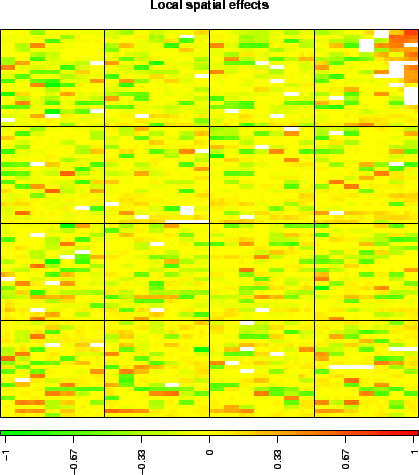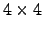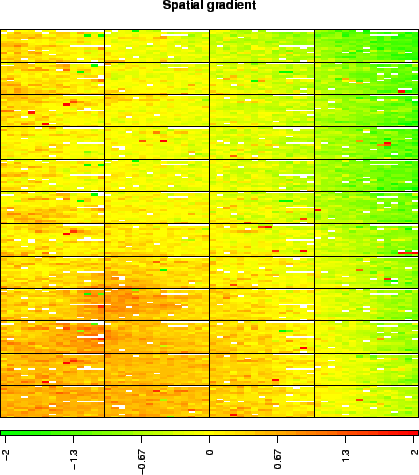Subsections

# 5 Data

We provide examples of array-CGH data coming from two different platforms. These data illustrate the need for appropriate within-array normalization methods, and especially the need for methods that handle spatial effects.

> data(spatial)


For each array we provide raw data (generated by Genepix or SPOT (5)), as well as the corresponding arrayCGH object before and after normalization.

These arrays illustrate the main source of non biological variability of these data sets, namely spatial effects. We classify these effects into two non exclusive types: local bias and global gradients. In the case of local bias, entire areas of the array show lower or higher signal values than the rest of the array, with no biological explanation (array edge); to our experience, this particular type of artifact roughly affects an array out of two. In the case of global gradients, the array shows an obvious signal gradient from one side of the slide to the other (array gradient).

## 5.1 edge

Bladder cancer tumors were collected at Henri Mondor Hospital (Créteil, France) (1) and hybridized on arrays CGH composed of 2464 Bacterian Artificial Chromosomes (F. Radvanyi, D. Pinkel et al., unpublished results); each of these BAC is spotted three times on the array, and the three replicates are neighbors on the array. We give the example of an arrayCGH with local spatial effects (figure 1): high log-ratios cluster in the upper-right corner of the array.

 > data(spatial) > arrayPlot(edge, "LogRatio", main = "Local spatial effects", zlim = c(-1, + 1), mediancenter = TRUE, bar = "h")We give the example of two arrays from a breast cancer data set from Institut Curie (O. Delattre, A. Aurias et al., unpublished results). These arrays consist of 3342 clones, organized as asuperblock that is replicated three times. This data set is affected by the two types of spatial effects: local bias areas (as for the previous data set), and spatial gradients from one side of the array to the other. The array gradient illustrates this second type of spatial effect.
 > data(spatial) > arrayPlot(gradient, "LogRatio", main = "Spatial gradient", zlim = c(-2, + 2), mediancenter = TRUE, bar = "h")